Ex 12.3

Chapter 12 Class 10 Areas related to Circles
Serial order wise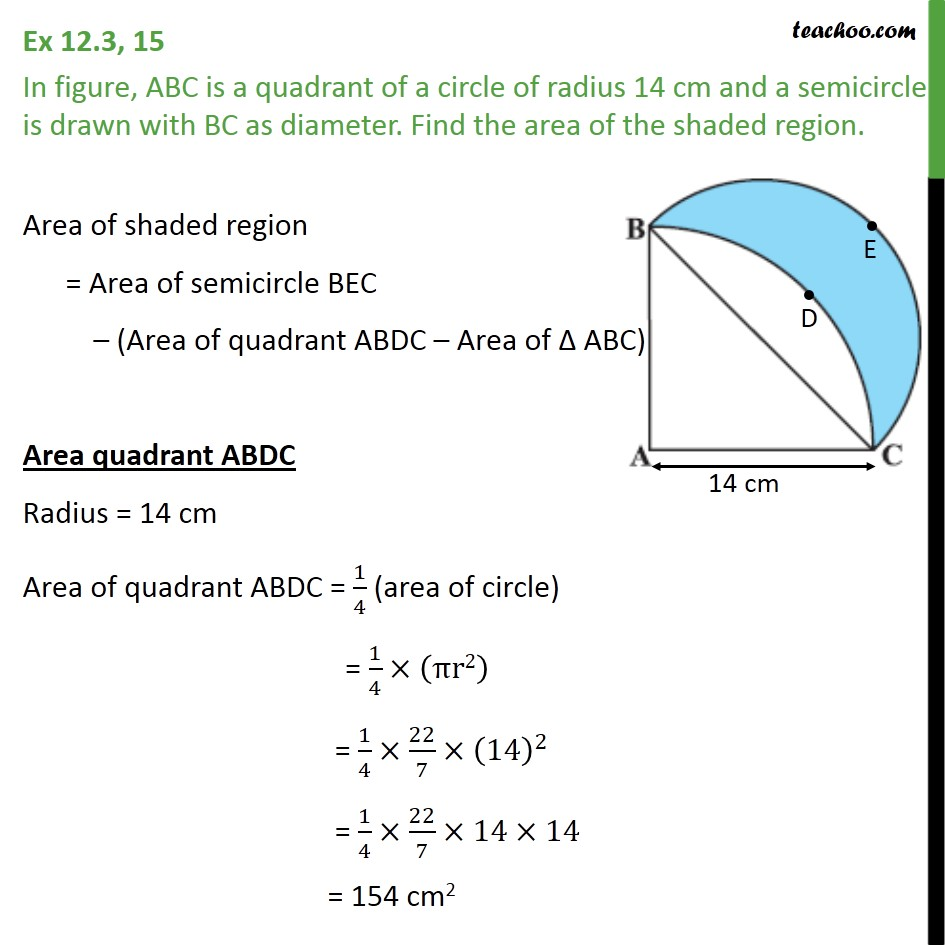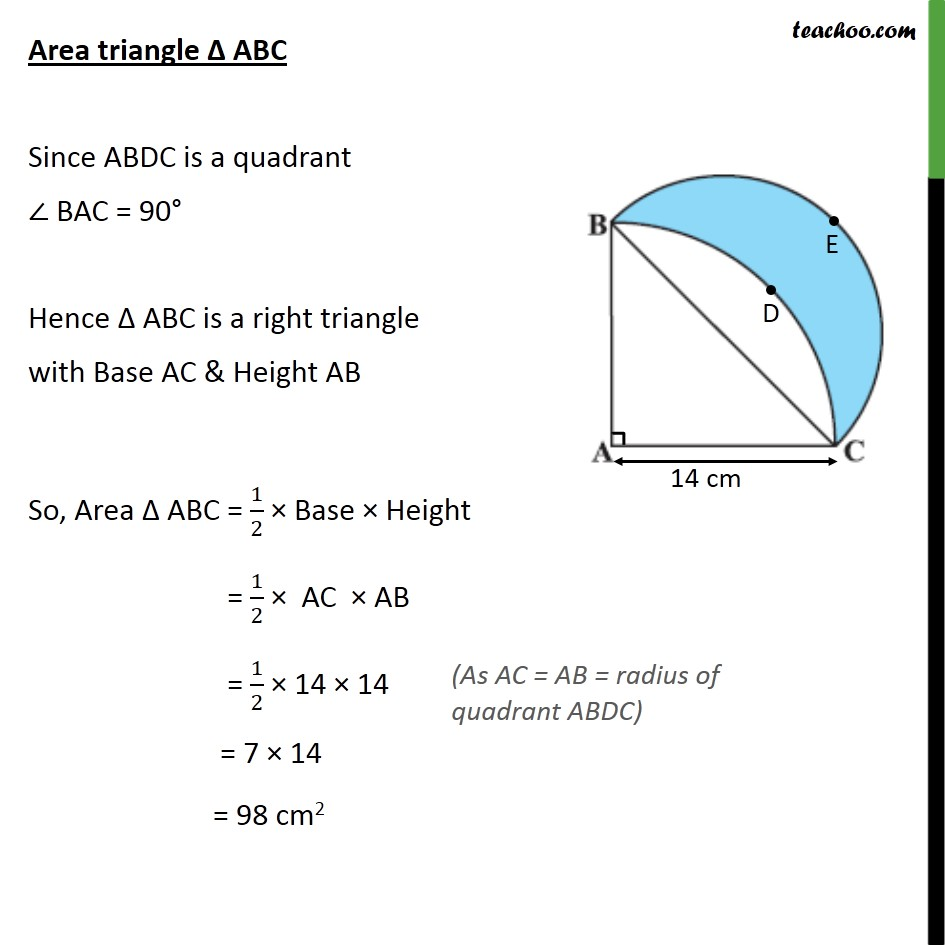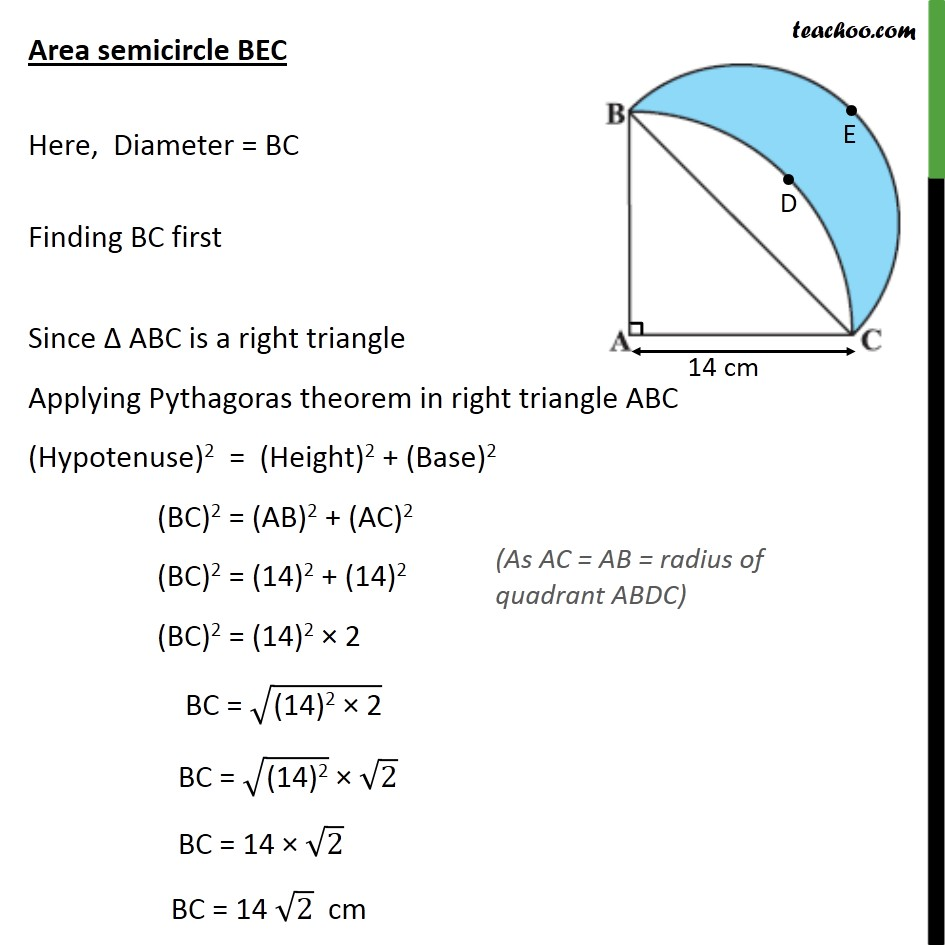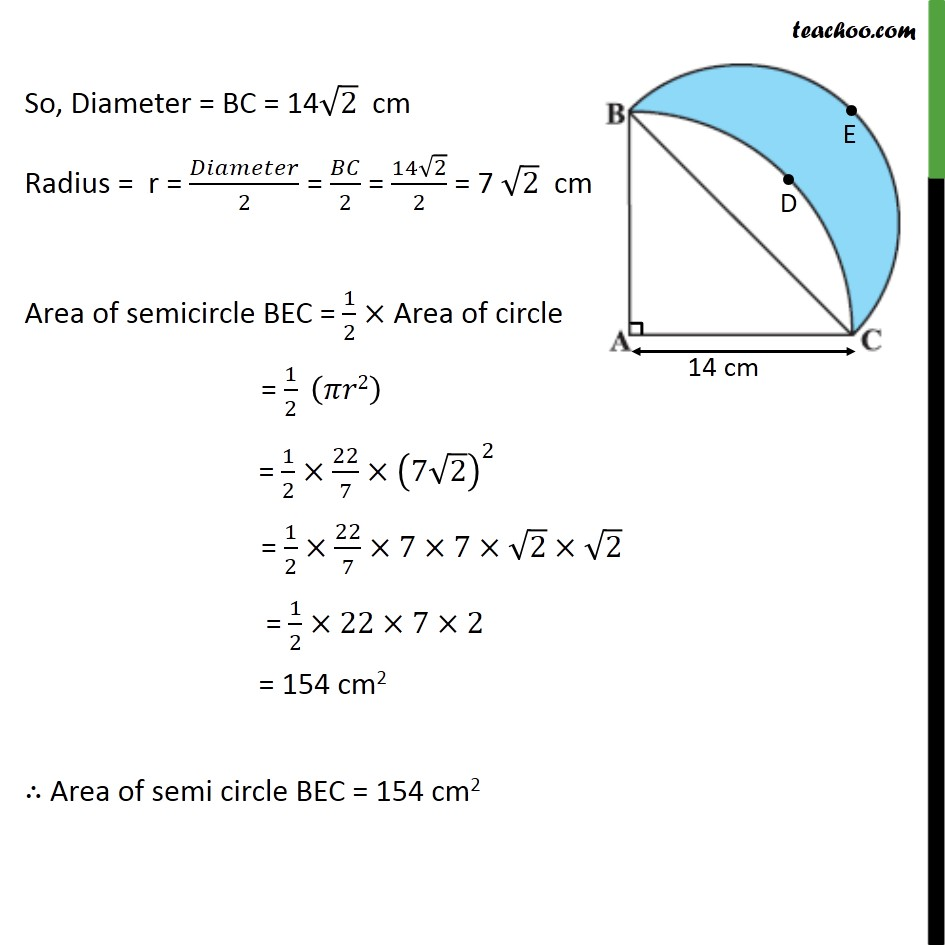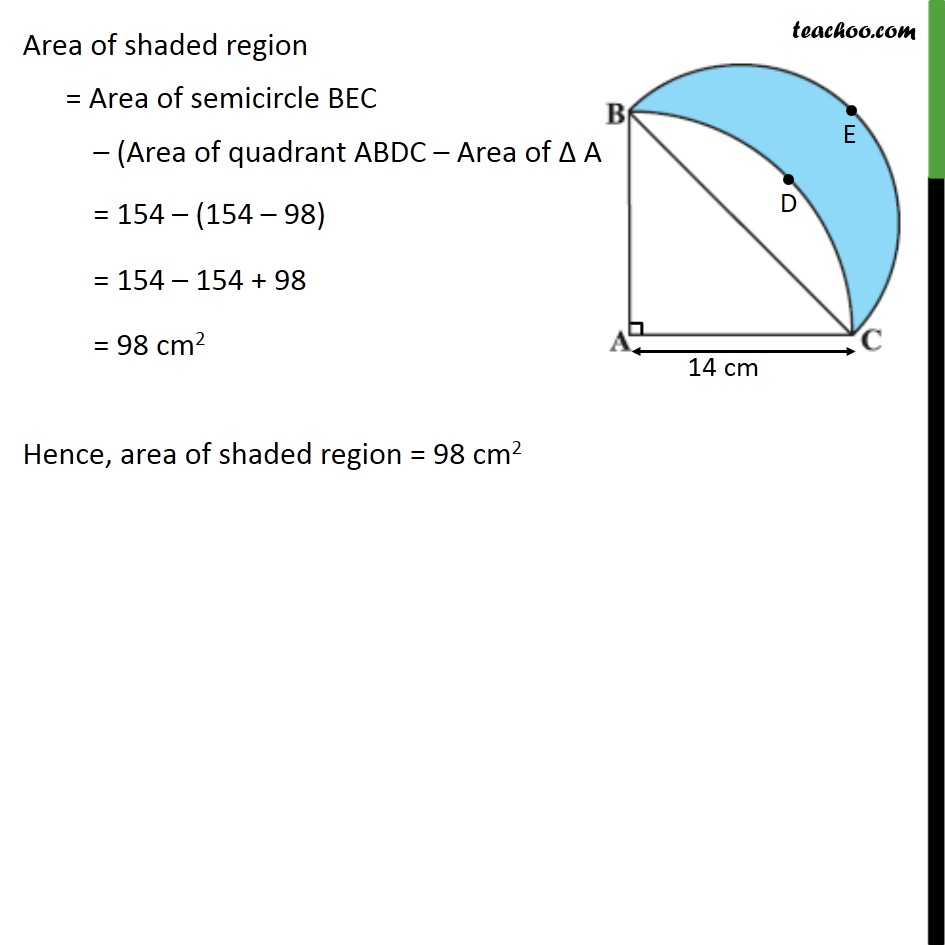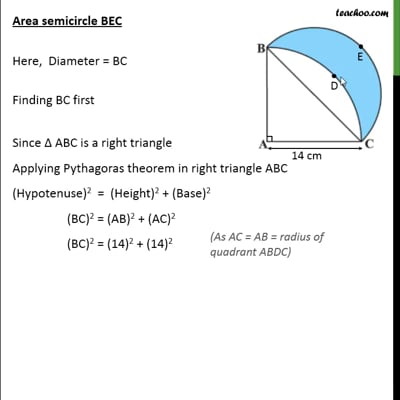This video is only available for Teachoo black users

Solve all your doubts with Teachoo Black (new monthly pack available now!)

### Transcript

Ex 12.3, 15 In figure, ABC is a quadrant of a circle of radius 14 cm and a semicircle is drawn with BC as diameter. Find the area of the shaded region. Area of shaded region = Area of semicircle BEC (Area of quadrant ABDC Area of ABC) Area quadrant ABDC Radius = 14 cm Area of quadrant ABDC = 1/4 (area of circle) = 1/4 ( r2) = 1/4 22/7 (14)^2 = 1/4 22/7 14 14 = 154 cm2 Area triangle ABC Since ABDC is a quadrant BAC = 90 Hence ABC is a right triangle with Base AC & Height AB So, Area ABC = 1/2 Base Height = 1/2 AC AB = 1/2 14 14 = 7 14 = 98 cm2 Area semicircle BEC Here, Diameter = BC Finding BC first Since ABC is a right triangle Applying Pythagoras theorem in right triangle ABC (Hypotenuse)2 = (Height)2 + (Base)2 (BC)2 = (AB)2 + (AC)2 (BC)2 = (14)2 + (14)2 (BC)2 = (14)2 2 BC = ("(14)2 2" ) BC = ("(14)2" ) ("2" ) BC = 14 ("2" ) BC = 14 2 cm So, Diameter = BC = 14 2 cm Radius = r = /2 = /2 = (14 2)/2 = 7 2 cm Area of semicircle BEC = 1/2 Area of circle = 1/2 ( 2) = 1/2 22/7 (7 2)^2 = 1/2 22/7 7 7 2 2 = 1/2 22 7 2 = 154 cm2 Area of semi circle BEC = 154 cm2 Area of shaded region = Area of semicircle BEC (Area of quadrant ABDC Area of ABC) = 154 (154 98) = 154 154 + 98 = 98 cm2 Hence, area of shaded region = 98 cm2